# Extend Line up to polygon feature

6485
16
04-14-2017 09:52 AMNew Contributor III

Hi  everyone,

I've some set of points which I'm joining to make a line. Refer the Picture below.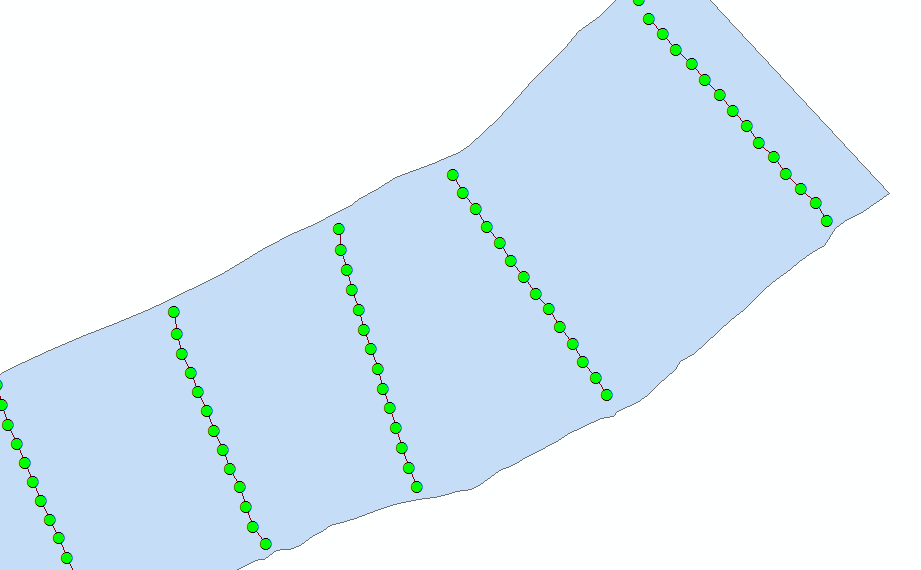The problem I'm facing is there are no points(elevation points) on the polygon (River Bank). Is there any way of extending the line to the polygon?

At present m using Extend line to layer, but it taking too much of time and inconsistent in the lines. Refer the Picture below.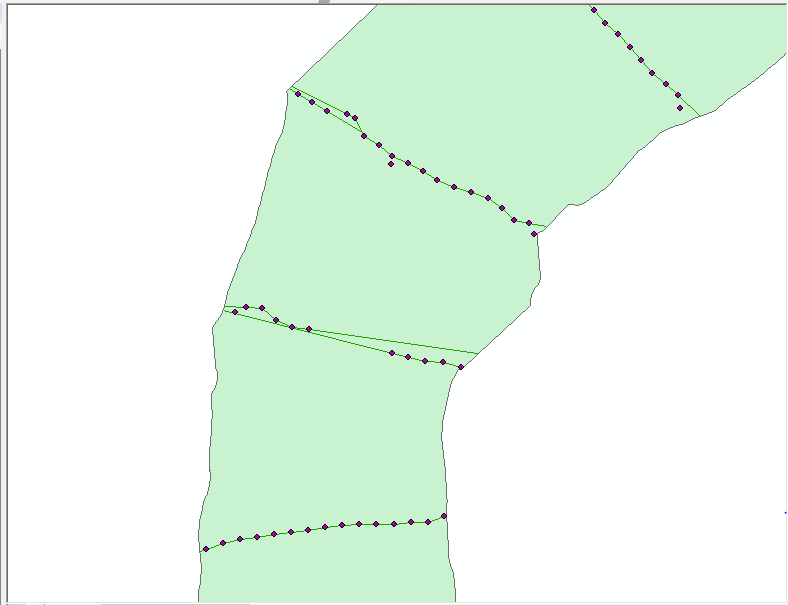Tags (4)
1 Solution

Accepted SolutionsbyMVP Esteemed Contributor

I just downloaded the sample data, and ran my code from the Draw a Line to the nearest Polygon thread, and it ran without any errors and did exactly what you wanted.  I did have to increase the dist value since you are using State Plane, and the gaps between the lines and river banks are greater than 100 feet.

Here is the code that worked for me, using your 2 shape files (load the shape files into ArcMap first and run from interactive Python window):

``````>>> import math
>>> dist = 500
>>>
>>> fc_line = "Pointoline"
>>> fc_poly = "RB_23"

>>> with arcpy.da.SearchCursor(fc_poly, "SHAPE@") as scur:
...     for poly, in scur:
...         boundary = poly.boundary()
...         arcpy.SelectLayerByLocation_management(fc_line, "WITHIN", poly)
...         with arcpy.da.UpdateCursor(fc_line, "SHAPE@") as ucur:
...             for line, in ucur:
...                 arr, = line.getPart()
...                 SR = line.spatialReference
...
...                 p1, p2 = arr[:2]
...                 angle = math.atan2(p2.Y - p1.Y, p2.X - p1.X)
...                 p = arcpy.Point(p1.X - dist * math.cos(angle),
...                                 p1.Y - dist * math.sin(angle))
...                 arr.insert(0, p)
...
...                 pn1, pn = arr[-2:]
...                 angle = math.atan2(pn.Y - pn1.Y, pn.X - pn1.X)
...                 p = arcpy.Point(pn.X + dist * math.cos(angle),
...                                 pn.Y + dist * math.sin(angle))
...                 arr.append(p)
...
...                 line = arcpy.Polyline(arr, SR)
...                 line = line.cut(boundary)
...                 ucur.updateRow([line])
...
>>>‍‍‍‍‍‍‍‍‍‍‍‍‍‍‍‍‍‍‍‍‍‍‍‍‍‍‍‍‍‍‍‍``````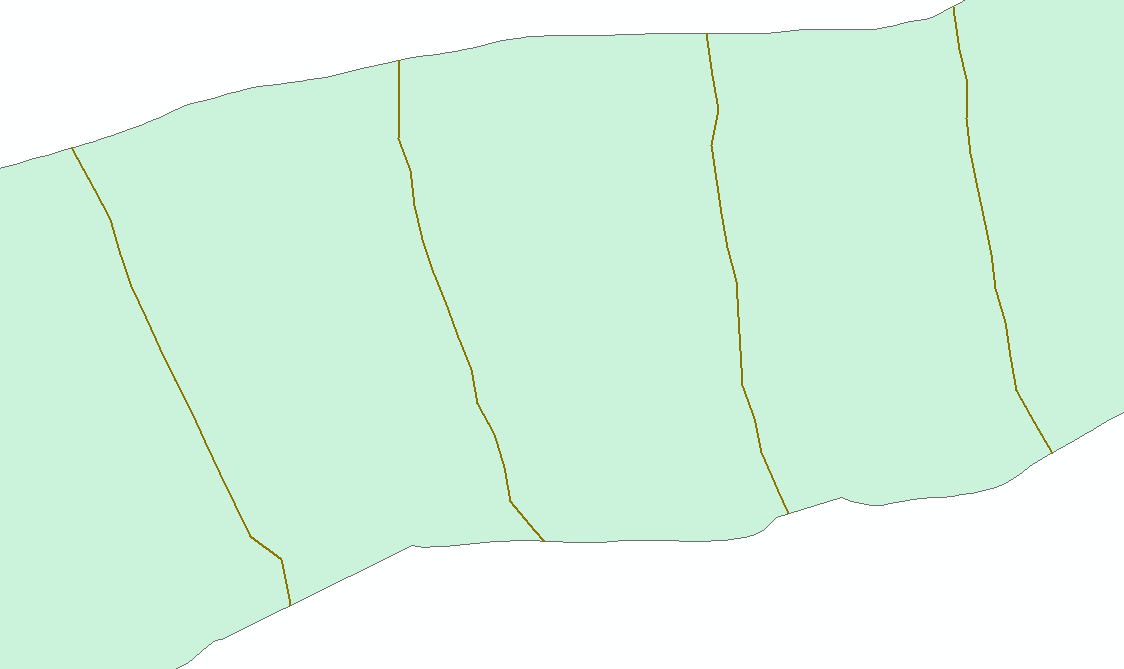16 RepliesbyEsri Esteemed Contributor

Can you share a sample of your data (points, lines and polygon)? The situations in the first image do not correspond to the situations in the second image (probably to do with the sequence of the points) and it would be good to know what is going on. I see some similarity with this thread Points to line in Arcmap , but you have already defined a way to connect the points into a line. There are several ways of extending the line to the river banks:

• extending the first and last vertex pair (using the same direction as defined by the vertex pairs)
• simply snapping the first and last points to the nearest point (edge) on the river bank

Both are highly depend on the "quality" of the input line. So if the line was created correctly, this should not be a problem. However the results from your second image suggest differently.byMVP Esteemed Contributor

I agree with Xander's comments and link.  I haven't used the ArcScript tool you mentioned, so I'm not sure what is too slow,  but you may want to look into using Map Topology to help you find the error, including the part of changing multiple issues at once.  But unless you do know that all the lines are geometrically clean, you will want to be carefully on doing anything in bulk.  You may want to do smaller batches so you can make sure other issues are not introduced.New Contributor III

Any python script that fixing the topological errors. Need in automated process. Have a huge amount of data for study. So, it difficult to run this tool manual.

Thanks for help.New Contributor III

Yes, I checked the Point to line . Actually, Same data I am also working for creating a line. We want to create a line feature from elevation point data. That line should touch the river banks all this we need automated process. The Second image which I am using the Extend line to layer tool for extend the output which got from the point to line tool. But Its taking time for 200 feature and getting geometrically errors.

Do you have any idea or script that create a line with the elevation point and line extend up to river banks.

Thanks for help.byMVP Esteemed ContributorNew Contributor III

Nothing was different but getting error with the script. Please find the sample data,byMVP Esteemed Contributor

I just downloaded the sample data, and ran my code from the Draw a Line to the nearest Polygon thread, and it ran without any errors and did exactly what you wanted.  I did have to increase the dist value since you are using State Plane, and the gaps between the lines and river banks are greater than 100 feet.

Here is the code that worked for me, using your 2 shape files (load the shape files into ArcMap first and run from interactive Python window):

``````>>> import math
>>> dist = 500
>>>
>>> fc_line = "Pointoline"
>>> fc_poly = "RB_23"

>>> with arcpy.da.SearchCursor(fc_poly, "SHAPE@") as scur:
...     for poly, in scur:
...         boundary = poly.boundary()
...         arcpy.SelectLayerByLocation_management(fc_line, "WITHIN", poly)
...         with arcpy.da.UpdateCursor(fc_line, "SHAPE@") as ucur:
...             for line, in ucur:
...                 arr, = line.getPart()
...                 SR = line.spatialReference
...
...                 p1, p2 = arr[:2]
...                 angle = math.atan2(p2.Y - p1.Y, p2.X - p1.X)
...                 p = arcpy.Point(p1.X - dist * math.cos(angle),
...                                 p1.Y - dist * math.sin(angle))
...                 arr.insert(0, p)
...
...                 pn1, pn = arr[-2:]
...                 angle = math.atan2(pn.Y - pn1.Y, pn.X - pn1.X)
...                 p = arcpy.Point(pn.X + dist * math.cos(angle),
...                                 pn.Y + dist * math.sin(angle))
...                 arr.append(p)
...
...                 line = arcpy.Polyline(arr, SR)
...                 line = line.cut(boundary)
...                 ucur.updateRow([line])
...
>>>‍‍‍‍‍‍‍‍‍‍‍‍‍‍‍‍‍‍‍‍‍‍‍‍‍‍‍‍‍‍‍‍``````byEsri Esteemed Contributor

The option provided by Joshua Bixby is a very good one. It takes the direction of the first and last part of the line and extends it to the polygon boundary. As an alternative you can simply snap the first and last point to polygon boundary (see code below).

``````#-------------------------------------------------------------------------------
# Name:       snapline2polygon.py
# Purpose:
#
# Author:      xbakker
#
# Created:     17/04/2017
#-------------------------------------------------------------------------------

def main():
print "Start process..."
import arcpy
fc_line = r'C:\GeoNet\SnapLine2Polygon\Pointoline.shp'
fc_pol = r'C:\GeoNet\SnapLine2Polygon\RB_23.shp'
fc_out = r'C:\GeoNet\SnapLine2Polygon\snapped_lines.shp'

# fech polygon and get perimeter
print "Get polygon and boundary"
polygon = arcpy.da.SearchCursor(fc_pol, ('SHAPE@')).next()
boundary = polygon.boundary()

print "start loop..."
lst_feats = []
cnt = 0
with arcpy.da.SearchCursor(fc_line, ('SHAPE@')) as curs:
for row in curs:
cnt += 1
if cnt % 10 == 0:
print " - processing line", cnt
polyline = row
pnt1 = polyline.firstPoint
pnt2 = polyline.lastPoint

pntg1_snap = boundary.snapToLine(pnt1)
pntg2_snap = boundary.snapToLine(pnt2)
polyline_out = ExtendPolyline(polyline, pntg1_snap.firstPoint, pntg2_snap.firstPoint)

lst_feats.append(polyline_out)

# store results
print "store results..."
arcpy.CopyFeatures_management(lst_feats, fc_out)

def ExtendPolyline(polyline, pnt1, pnt2):
# will snap first and last line to nearest part of given polyline
# does not account for small segments!
sr = polyline.spatialReference
lst_pnts = []
for part in polyline:
for pnt in part:
lst_pnts.append(pnt)

lst_pnts.insert(0, pnt1)
lst_pnts.append(pnt2)
return arcpy.Polyline(arcpy.Array(lst_pnts), sr)

if __name__ == '__main__':
main()
‍‍‍‍‍‍‍‍‍‍‍‍‍‍‍‍‍‍‍‍‍‍‍‍‍‍‍‍‍‍‍‍‍‍‍‍‍‍‍‍‍‍‍‍‍‍‍‍‍‍‍‍‍‍‍‍‍‍‍‍‍‍‍‍‍‍‍‍‍‍‍‍‍‍‍‍‍‍‍‍‍‍‍‍‍‍‍‍‍‍‍‍‍‍‍‍‍‍‍‍‍‍‍‍‍‍‍‍‍‍‍‍‍‍‍‍‍‍‍‍‍``````

This will produce errors when you have small segments and the first of last point is closer to the wrong side of the polygon boundary: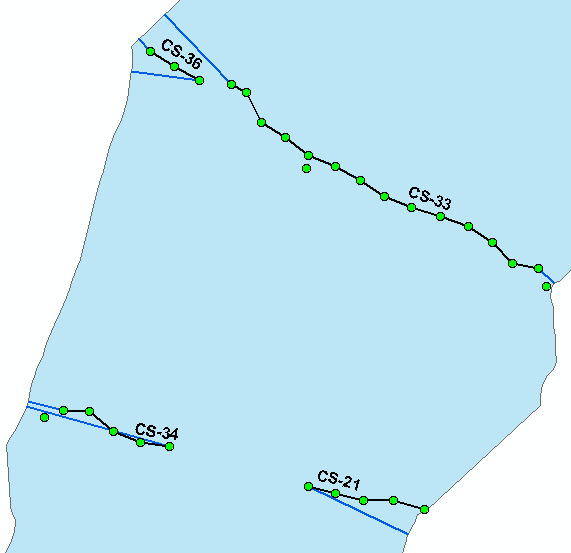To avoid this you would have to edit the line data and create the correct lines to be extended or use the logic suggested by Joshua.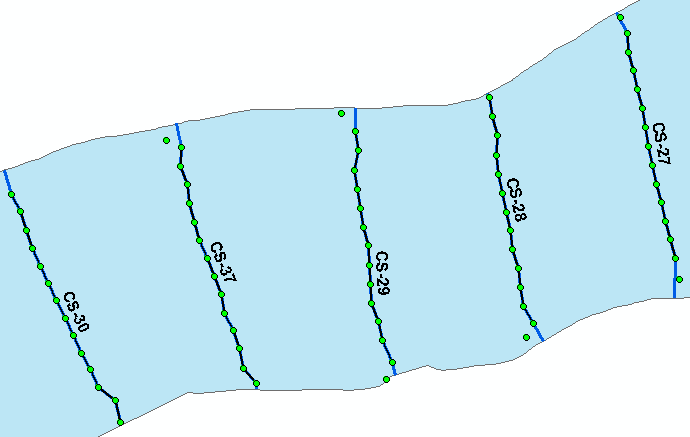New Contributor III

But, I am getting error at p1,p2 = arr[:2] and using arcmap 10.3

Runtime error
Traceback (most recent call last):
File "<string>", line 10, in <module>
File "c:\program files (x86)\arcgis\desktop10.3\arcpy\arcpy\arcobjects\mixins.py", line 161, in __getitem__
return convertArcObjectToPythonObject(self._arc_object.GetObject(index))
AttributeError: Array: Error in parsing arguments for GetObject

Thanks for help.